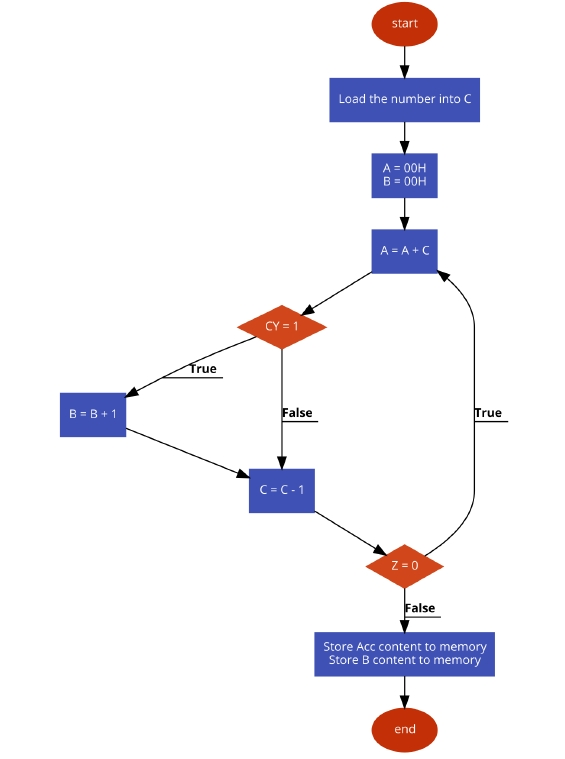# 8085 program to find the sum of first n natural numbers

In this program we will see how to add first n natural numbers.

### Problem Statement

Write 8085 Assembly language program to add first N natural numbers. The value of N is provided.

### Discussion

We are getting the value of N from memory location 8000H. We are using the number N as count variable, in each step we are calculating (A + Count) value, and store them into A. After adding them, the count value is decreased,thus the total series is completed.

If the number is 23H(35D), then the sum will be (35*36)/2 = 630 (276H)

Data
.
.
.
.
.
.
8000
23
.
.
.
.
.
.

### Flow Diagram### Program

HEX Codes
Labels
Mnemonics
F000
21, 00, 80

LXI H,8000H
Point to get the upper limit
F003
4E

MOV C, M
Load the upper limit to C
F004
AF

XRA A
Clear the A register
F005
47

MOV B, A
Also clear B register
F006
81
LOOP
F007
D2, 0B, F0

JNC SKIP
If CY = 0,Skip next step
F00A
04

INR B
Increase B ifCY = 1
F00B
0D
SKIP
DCR C
Decrease C by1
F00C
C2, 06, F0

JNZ LOOP
Until Z = 1,go to LOOP
F00F
21, 50, 80

LXI H, 8050H
F012
77

MOV M, A
Store the acc content
F013
23

INX H
Point to next location
F014
70

MOV M, B
Store the MSbyte
F015
76

HLT
Terminate the program

Data
.
.
.
.
.
.
8050
76
8051
02
.
.
.
.
.
.